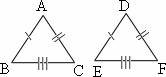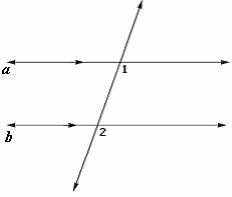## Definition Of Postulate

Postulate is a true statement, which does not require to be proved.

Postulate is used to derive the other logical statements to solve a problem.
Postulates are also called as axioms.

### Example of PostulateTo prove that these triangles are congruent, we use SSS postulate, as the corresponding sides of both the triangles are equal.

### Solved Example on Postulate

#### Ques: State the postulate or theorem you would use to prove that ∠1 and ∠2 are congruent.##### Choices:

A. corresponding angles postulate
B. converse of corresponding angles postulate
C. alternate angles are congruent